# Function Recursion to understand recursion you must understand

• Slides: 8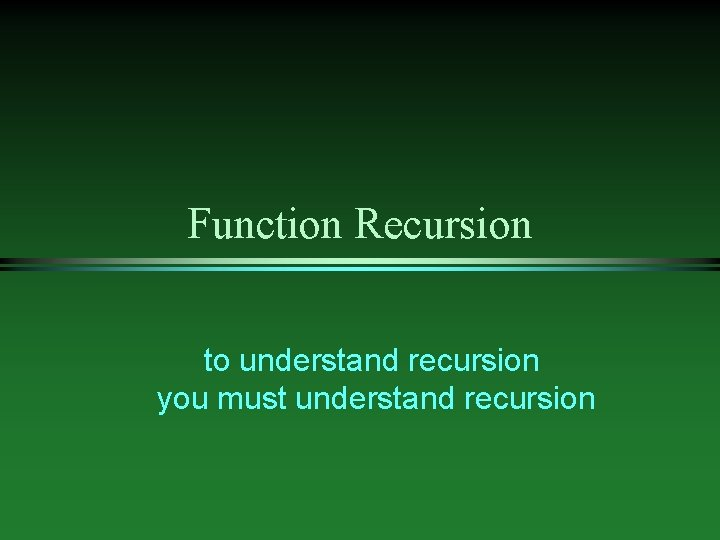Function Recursion to understand recursion you must understand recursion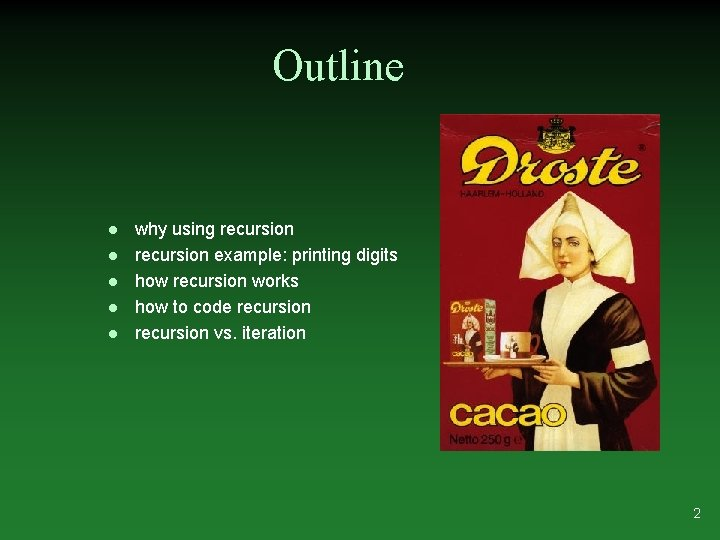Outline l l l why using recursion example: printing digits how recursion works how to code recursion vs. iteration 2Why Recursion l l l in top-down design one of the major techniques is to break the task into subtasks and code the tasks separately it may turn out that one of the subtasks is a smaller version of the larger task example: to search an array you split it into halves, searching each half is similar to searching the whole array can be solved with recursion recursive function definition – the definition contains calls to itself 3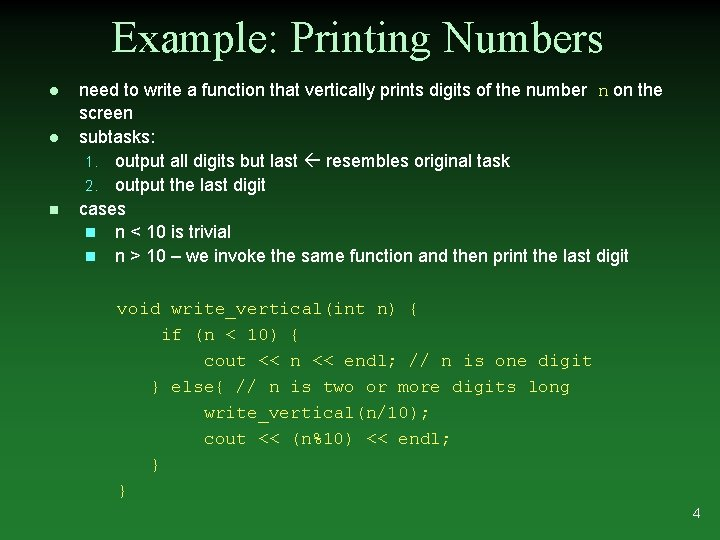Example: Printing Numbers l l n need to write a function that vertically prints digits of the number n on the screen subtasks: 1. output all digits but last resembles original task 2. output the last digit cases n n < 10 is trivial n n > 10 – we invoke the same function and then print the last digit void write_vertical(int n) { if (n < 10) { cout << n << endl; // n is one digit } else{ // n is two or more digits long write_vertical(n/10); cout << (n%10) << endl; } } 4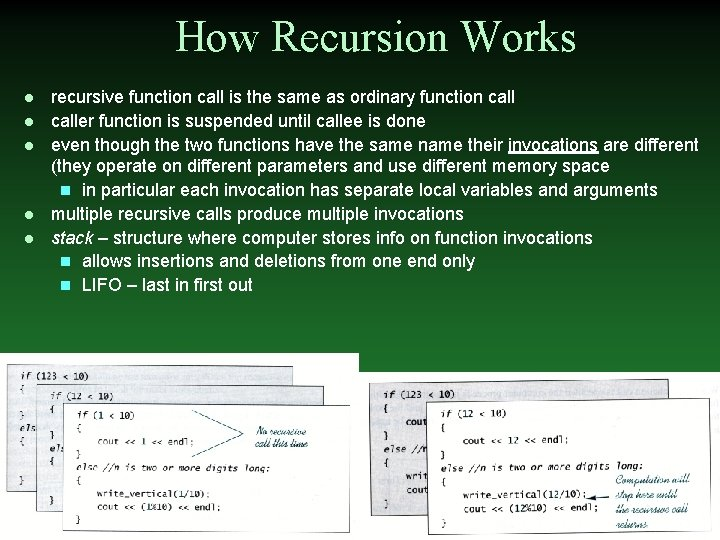How Recursion Works l l l recursive function call is the same as ordinary function caller function is suspended until callee is done even though the two functions have the same name their invocations are different (they operate on different parameters and use different memory space n in particular each invocation has separate local variables and arguments multiple recursive calls produce multiple invocations stack – structure where computer stores info on function invocations n allows insertions and deletions from one end only n LIFO – last in first out 5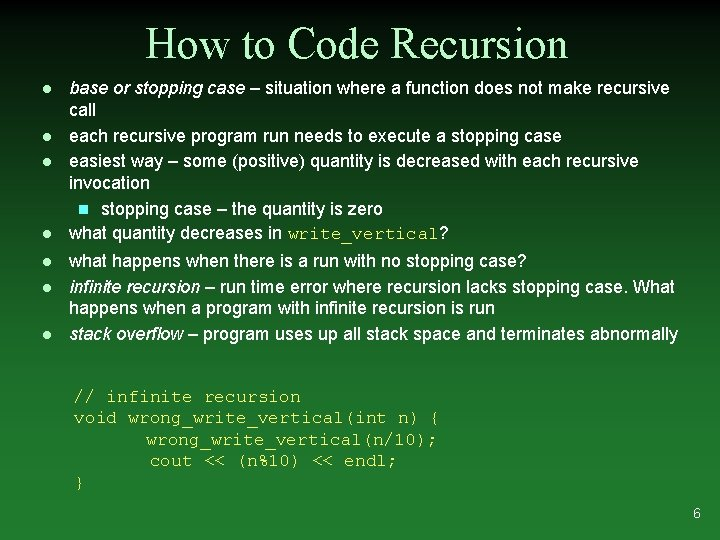How to Code Recursion l l l l base or stopping case – situation where a function does not make recursive call each recursive program run needs to execute a stopping case easiest way – some (positive) quantity is decreased with each recursive invocation n stopping case – the quantity is zero what quantity decreases in write_vertical? what happens when there is a run with no stopping case? infinite recursion – run time error where recursion lacks stopping case. What happens when a program with infinite recursion is run stack overflow – program uses up all stack space and terminates abnormally // infinite recursion void wrong_write_vertical(int n) { wrong_write_vertical(n/10); cout << (n%10) << endl; } 6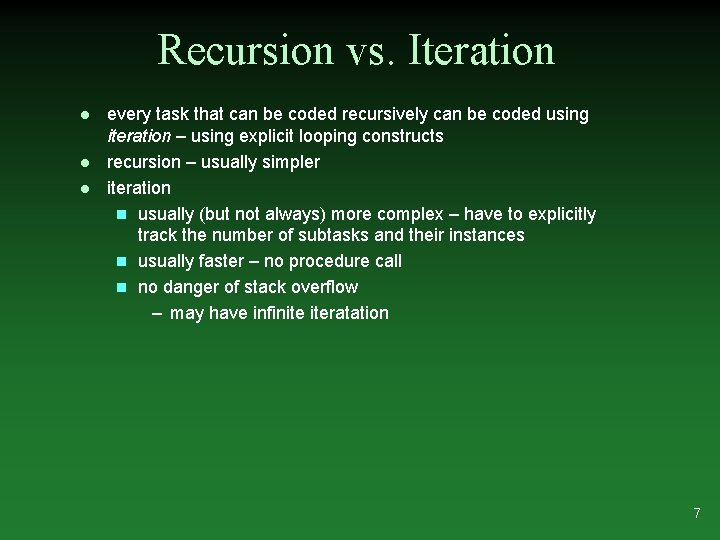Recursion vs. Iteration l l l every task that can be coded recursively can be coded using iteration – using explicit looping constructs recursion – usually simpler iteration n usually (but not always) more complex – have to explicitly track the number of subtasks and their instances n usually faster – no procedure call n no danger of stack overflow – may have infinite iteratation 7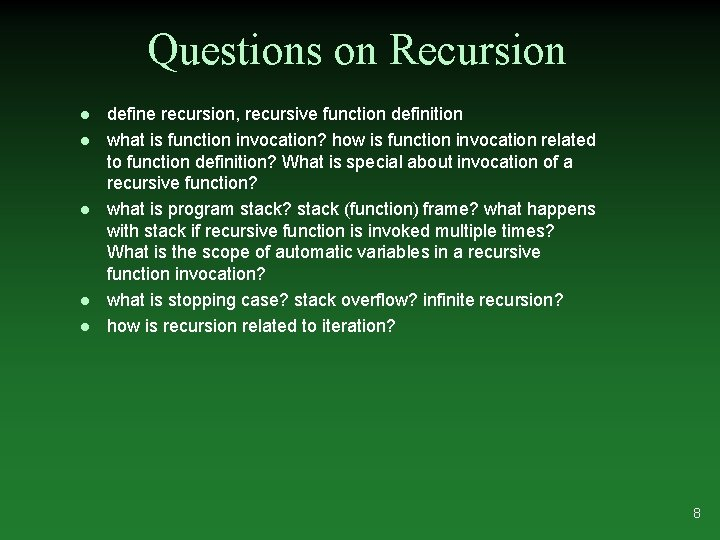Questions on Recursion l l l define recursion, recursive function definition what is function invocation? how is function invocation related to function definition? What is special about invocation of a recursive function? what is program stack? stack (function) frame? what happens with stack if recursive function is invoked multiple times? What is the scope of automatic variables in a recursive function invocation? what is stopping case? stack overflow? infinite recursion? how is recursion related to iteration? 8